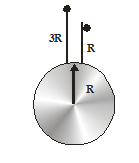Gravitaional potential energy
Question

# A particle is dropped from height 3R from surface of earth. Then, the velocity of particle at height R is (given R is radius of earth, g is acceleration due to gravity on the earth’s surface) :

Moderate
Solution

## Principle of conservation of energy is to be applied. Since acceleration is variable $\begin{array}{l}-\frac{\mathrm{GmM}}{4\mathrm{R}}=-\frac{\mathrm{GmM}}{2\mathrm{R}}+\frac{1}{2}{\mathrm{mv}}^{2}\\ \mathrm{v}=\sqrt{\frac{\mathrm{GM}}{2\mathrm{R}}}=\sqrt{\frac{\mathrm{gR}}{2}}\end{array}$Get Instant Solutions